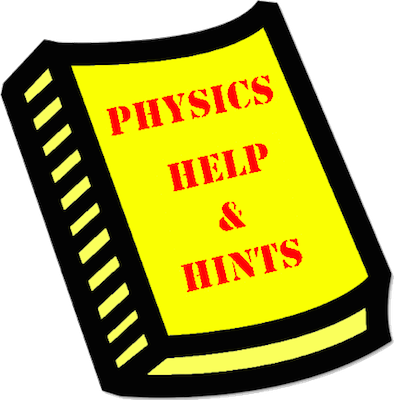# Mission CG5: Mathematical Analysis of Circular Motion

Mission CG5 consists of eight physical situations involving the motion of an object in a circle. You will have to combine an understanding of force types, free-body diagrams, and Fnet = m•a to analyze the situation. The mission consists of 32 questions organized into 8 Question Groups. You must answer one question from each Question Group to complete the mission. The learning outcomes for this mission are ...

#### Learning Outcomes

• The student should be able to utilize Newton's laws to analyze the motion of an object moving in a horizontal circle and to determine the values of the acceleration, net force and individual forces.
• The student should be able utilize Newton's laws to analyze the motion of an object moving in a vertical circle and to determine the values of the acceleration, net force and individual forces.

### Launch Mission CG5

#### Getting Help

If you are not familiar with this topic, then you should first learn about the topic using our written Tutorial or our Video Tutorial:

The Physics Classroom - Circular Motion Unit, Lesson 1, Part e
The Physics Classroom - Circular Motion Unit, Lesson 2, Part a

####Question-Specific Help

Each Question Group has its own Help page with information specific to the question. You can access the Help page from within the mission by tapping on the Help Me! icon (textbook). For your convenience, links to those pages are provided below: